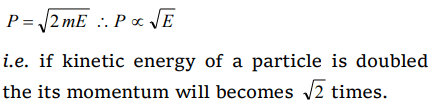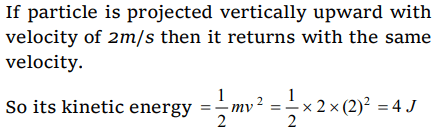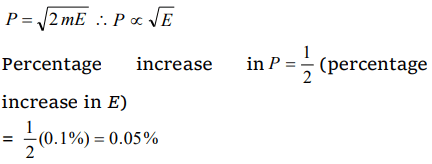## Work, Energy and Power Questions and Answers Part-9

1.If the K.E. of a particle is doubled, then its momentum will
a) Remain unchanged
b) Be doubled
d) Increase $\sqrt{2}$ times

Explanation:2. If the stone is thrown up vertically and return to ground, its potential energy is maximum
a) During the upward journey
b) At the maximum height
c) During the return journey
d) At the bottom

Explanation: Potential energy = mgh
Potential energy is maximum when h is maximum

3. A body of mass 2 kg is projected vertically upwards with a velocity of $2 m s^{-1}$ . The K.E. of the body just before striking the ground is
a) 2 J
b) 1 J
c) 4 J
d) 8 J

Explanation:4. The energy stored in wound watch spring is
a) K.E
b) P.E
c) Heat energy
d) Chemical energy

Explanation: P.E

5. Two bodies of different masses $m_{1}$ and $m_{2}$ have equal momenta. Their kinetic energies $E_{1}$ and $E_{2}$ are in the ratio
a) $\sqrt{m_{1}}:\sqrt{m_{2}}$
b) $m_{1}:m_{2}$
c) $m_{2}:m_{1}$
d) $m_1^2:m_2^2$

Explanation:6.A car travelling at a speed of 30 km/hour is brought to a halt in 8 m by applying brakes. If the same car is travelling at 60 km/hour, it can be brought to a halt with the same braking force in
a) 8 m
b) 16 m
c) 24 m
d) 32 m

Explanation: s $\propto$ u2 i.e. if speed becomes double then stopping distance will become four times i.e. 8 * 4 = 32m

7. Tripling the speed of the motor car multiplies the distance needed for stopping it by,
a) 3
b) 6
c) 9
d) Some other number

Explanation: s $\propto$ u2 i.e. if speed becomes three times then distance needed for stopping will be nine times

8. If the kinetic energy of a body increases by 0.1%, the percent increase of its momentum will be
a) 0.05%
b) 0.1%
c) 1.0%
d) 10%

Explanation:9. If velocity of a body is twice of previous velocity, then kinetic energy will become
a) 2 times
b) $\frac{1}{2}times$
c) 4 times
d) 1 timesd) $\sqrt{3}:1$